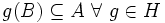Ping-pong lemma

Statement

Statement in terms of two elements and free groups

Suppose$G$ is a group with a group action on a set$X$. Further, suppose$A$ and$B$ are two subsets of$X$ such that neither is contained in the other. Further, suppose$a,b \in G$ are elements such that:

•$b^n(A) \subseteq B \ \forall \ n \ne 0$.
•$a^n(B) \subseteq A \ \forall \ n \ne 0$.

Then, the subgroup$\langle a, b \rangle$ is a free group with$\{a,b\}$ as a freely generating set.

Statement in terms of two subgroups and free products

Suppose$G$ is a group with a group action on a set$X$. Further, suppose$A$ and$B$ are two subsets of$X$ such that neither is contained in the other. Further, suppose$H, K \le G$ are subgroups such that:

•$g(A) \subseteq B \ \forall \ g \in K$.
•$g(B) \subseteq A \ \forall \ g \in H$.

Then, the subgroup$\langle H, K \rangle$ is an internal free product of the subgroups$H$ and$K$.